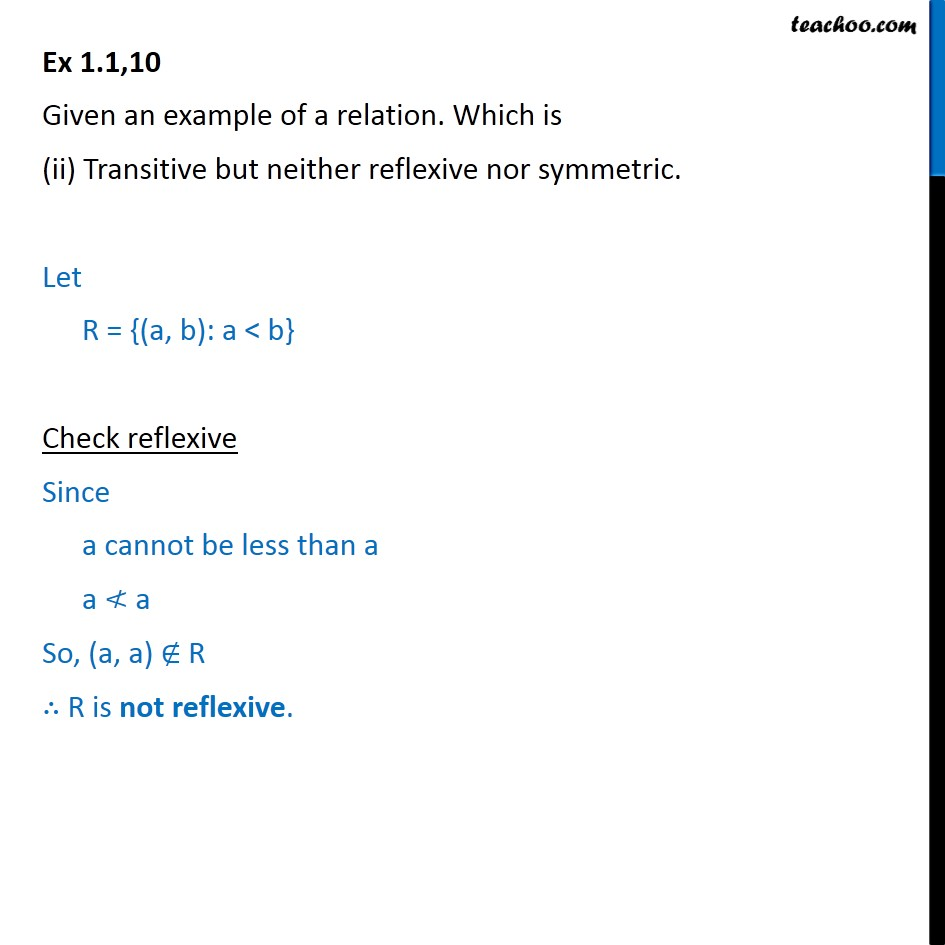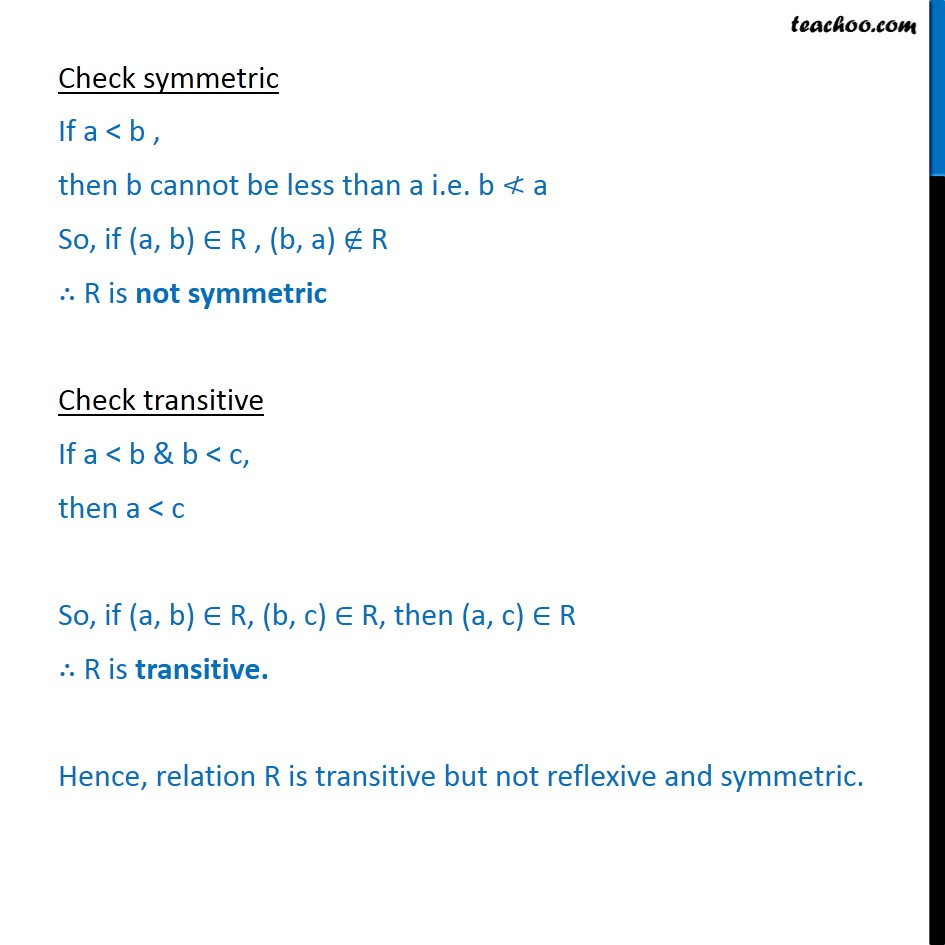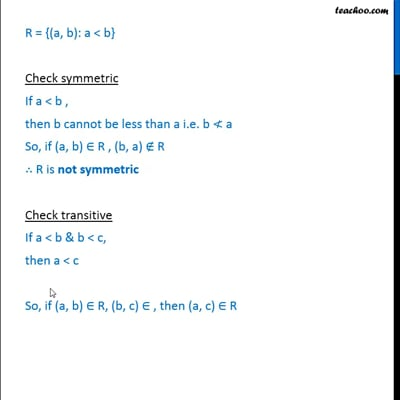Ex 1.1

Chapter 1 Class 12 Relation and Functions
Serial order wiseThis video is only available for Teachoo black users

Introducing your new favourite teacher - Teachoo Black, at only ₹83 per month

### Transcript

Ex 1.1,10 Given an example of a relation. Which is (ii) Transitive but neither reflexive nor symmetric. Let R = {(a, b): a < b} Check reflexive Since a cannot be less than a a a So, (a, a) R R is not reflexive. Check symmetric If a < b , then b cannot be less than a i.e. b a So, if (a, b) R , (b, a) R R is not symmetric Check transitive If a < b & b < c, then a < c So, if (a, b) R, (b, c) R, then (a, c) R R is transitive. Hence, relation R is transitive but not reflexive and symmetric.